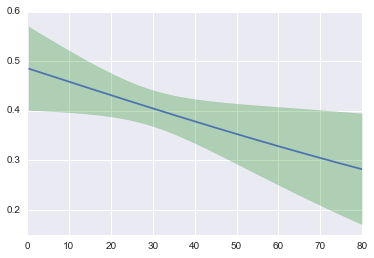In :
%matplotlib inline

import pandas as pd
import seaborn as sns
import statsmodels.api as sm
pd.options.display.max_rows = 10

In :
df = (sns.load_dataset('titanic')
.dropna(subset=['survived', 'age']))

Out:
survived pclass sex age sibsp parch fare embarked class who adult_male deck embark_town alive alone
0 0 3 male 22 1 0 7.2500 S Third man True NaN Southampton no False
1 1 1 female 38 1 0 71.2833 C First woman False C Cherbourg yes False
2 1 3 female 26 0 0 7.9250 S Third woman False NaN Southampton yes True
3 1 1 female 35 1 0 53.1000 S First woman False C Southampton yes False
4 0 3 male 35 0 0 8.0500 S Third man True NaN Southampton no True
In :
mod = sm.Logit.from_formula('survived ~ age', df)
res = mod.fit()
me = res.get_margeff()

Optimization terminated successfully.
Current function value: 0.672429
Iterations 4

In :
res.summary()

Out:
Dep. Variable: No. Observations: survived 714 Logit 712 MLE 1 Fri, 11 Sep 2015 0.004445 08:16:34 -480.11 True -482.26 0.03839
coef std err z P>|z| [0.025 0.975] -0.0567 0.174 -0.327 0.744 -0.397 0.283 -0.0110 0.005 -2.057 0.040 -0.021 -0.001
In :
me.summary()

Out:
Dep. Variable: survived dydx overall
dy/dx std err z P>|z| [95.0% Conf. Int.]
age -0.0026 0.001 -2.081 0.037 -0.005

Using Greene's notation here (~page 736). We've got a few things

• Exogenous: $X$, a single row is $x.T$
• estimated coef $\hat{\beta}$
• predicted prob $\hat{F} = \Lambda(\bf(x)^T\hat{\bf{\beta}})$ where $\Lambda$ is the logistic CDF
• Variance of $\hat{\beta} = V$, from statsmodels

We want a prediction interval for the predicted probability around the CEF. We have

$Asy. Var[\hat{F}] = [\partial \hat{F} / \partial \hat{\beta}]\bf{V} [\partial \hat{F} / \partial \hat{\beta}]$

And the vector of derivatives is

$[\partial \hat{F} / \partial \hat{\beta}] = [d\hat{F}/dz][\partial z / \partial \hat{\beta}] = \hat{f} x$

where $\hat{f} = \hat{\Lambda}(1 - \hat{\Lambda})$ (the logistic pdf I beleive).

And so

$Asy.Var[\hat{F}] = \hat{f}^2 x^T\bf{V}x$

Note that the $x$ here is a single row from the matrix. But vectors are always column vectors so $x$ is $Kx1$, where $K$ is the number of predictors (2 for us).

In :
from scipy import stats

Λ = lambda x: stats.logistic().cdf(x)
λ = lambda x: stats.logistic().pdf(x)

β_ = res.params.values.reshape(-1, 1)
V_ = res.cov_params().values

In :
# this is the equation from above for Var(F)
# only works for a single observation though
def var_π(x, β, V_):
#      λ(z)**s * x.T @ V_ @ x
prob = λ(x.T.dot(β))**2 * x.T.dot(V_).dot(x)  # maybe this is it?
return prob

In :
# for multiple observations, but not quite vectorized fully

def var_πs(xx, β, V_):
α = λ(xx.dot(β))**2
out = np.empty((500, 1))
for i, x in enumerate(xx):
out[i] = x.T.dot(V_).dot(x)
return α * out

In :
# Making some fake data.
xx = sm.add_constant(np.linspace(df.age.min(), df.age.max(), 500).reshape(-1, 1))
πs = Λ(xx.dot(β_))
vv = np.sqrt(var_πs(xx, β_, V_))

In :
plt.plot(xx[:, 1], πs)
plt.fill_between(xx[:, 1], (πs - 1.96*vv).ravel(), (πs + 1.96*vv).ravel(),
alpha=.25, color='g')

Out:
<matplotlib.collections.PolyCollection at 0x108b62438>In [ ]: